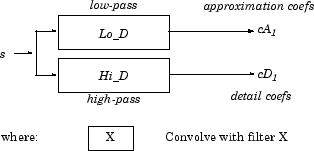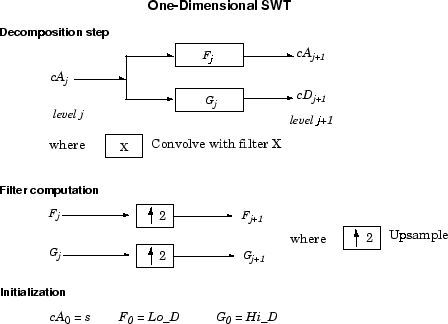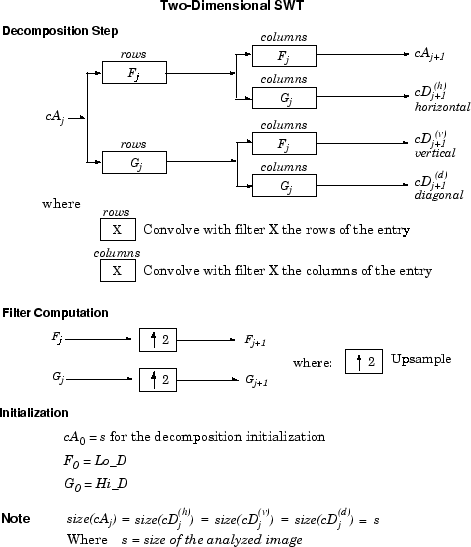## Nondecimated Discrete Stationary Wavelet Transforms (SWTs)

We know that the classical DWT suffers a drawback: the DWT is not a time-invariant transform. This means that, even with periodic signal extension, the DWT of a translated version of a signal X is not, in general, the translated version of the DWT of X.

How to restore the translation invariance, which is a desirable property lost by the classical DWT? The idea is to average some slightly different DWT, called ε-decimated DWT, to define the stationary wavelet transform (SWT). This property is useful for several applications such as breakdown points detection.

The main application of the SWT is denoising. For more information on the rationale, see [CoiD95] in References. For examples, see 1-D Stationary Wavelet Transform and 2-D Stationary Wavelet Transform.

The principle is to average several denoised signals. Each of them is obtained using the usual denoising scheme (see Wavelet Denoising and Nonparametric Function Estimation), but applied to the coefficients of an ε-decimated DWT.

Note

The SWT is defined only for signals of length divisible by 2J, where J is the maximum decomposition level. The SWT uses periodic (`per`) extension.

### ε-Decimated DWT

What is an ε-decimated DWT?

There exist a lot of slightly different ways to handle the discrete wavelet transform. Let us recall that the DWT basic computational step is a convolution followed by a decimation. The decimation retains even indexed elements.

But the decimation could be carried out by choosing odd indexed elements instead of even indexed elements. This choice concerns every step of the decomposition process, so at every level we chose odd or even.

If we perform all the different possible decompositions of the original signal, we have 2J different decompositions, for a given maximum level J.

Let us denote by εj = 1 or 0 the choice of odd or even indexed elements at step j. Every decomposition is labeled by a sequence of 0s and 1s: ε = ε1...,εJ. This transform is called the ε-decimated DWT.

You can obtain the basis vectors of the ε-decimated DWT from those of the standard DWT by applying a shift and corresponds to a special choice of the origin of the basis functions.

### How to Calculate the ε-Decimated DWT: SWT

It is possible to calculate all the ε-decimated DWT for a given signal of length N, by computing the approximation and detail coefficients for every possible sequence ε. Do this using iteratively, a slightly modified version of the basic step of the DWT of the form:

```[A,D] = dwt(X,wname,'mode','per','shift',e); ```

The last two arguments specify the way to perform the decimation step. This is the classical one for `e =` 0, but for `e =` 1 the odd indexed elements are retained by the decimation.

Of course, this is not a good way to calculate all the ε-decimated DWT, because many computations are performed many times. We shall now describe another way, which is the stationary wavelet transform (SWT).

The SWT algorithm is very simple and is close to the DWT one. More precisely, for level 1, all the ε-decimated DWT (only two at this level) for a given signal can be obtained by convolving the signal with the appropriate filters as in the DWT case but without downsampling. Then the approximation and detail coefficients at level 1 are both of size N, which is the signal length. This can be visualized in the following figure.The general step j convolves the approximation coefficients at level j–1, with upsampled versions of the appropriate original filters, to produce the approximation and detail coefficients at level j. This can be visualized in the following figure.Next, we illustrate how to extract a given ε-decimated DWT from the approximation and detail coefficients structure of the SWT.

We decompose a sequence of height numbers with the SWT, at level J = 3, using an orthogonal wavelet.

The function `swt` calculates successively the following arrays, where A(j,ε1,...,εj) or D(j,ε1,...,εj) denotes an approximation or a detail coefficient at level j obtained for the ε-decimated DWT characterized by ε=[ε1,...,εj].

#### Step 0 (Original Data)

 A(0) A(0) A(0) A(0) A(0) A(0) A(0) A(0)

#### Step 1

 D(1,0) D(1,1) D(1,0) D(1,1) D(1,0) D(1,1) D(1,0) D(1,1) A(1,0) A(1,1) A(1,0) A(1,1) A(1,0) A(1,1) A(1,0) A(1,1)

#### Step 2

 D(1,0) D(1,1) D(1,0) D(1,1) D(1,0) D(1,1) D(1,0) D(1,1) D(2,0,0) D(2,1,0) D(2,0,1) D(2,1,1) D(2,0,0) D(2,1,0) D(2,0,1) D(2,1,1) A(2,0,0) A(2,1,0) A(2,0,1) A(2,1,1) A(2,0,0) A(2,1,0) A(2,0,1) A(2,1,1)

#### Step 3

 D(1,0) D(1,1) D(1,0) D(1,1) D(1,0) D(1,1) D(1,0) D(1,1) D(2,0,0) D(2,1,0) D(2,0,1) D(2,1,1) D(2,0,0) D(2,1,0) D(2,0,1) D(2,1,1) D(3,0,0,0) D(3,1,0,0) D(3,0,1,0) D(3,1,1,0) D(3,0,0,1) D(3,1,0,1) D(3,0,1,1) D(3,1,1,1) A(3,0,0,0) A(3,1,0,0) A(3,0,1,0) A(3,1,1,0) A(3,0,0,1) A(3,1,0,1) A(3,0,1,1) A(3,1,1,1)

Let j denote the current level, where j is also the current step of the algorithm. Then we have the following abstract relations with εi = 0 or 1:

```[tmpAPP,tmpDET] = dwt(A(j,ε1, ,ɛj),wname,'mode','per','shift',ɛj+1); A(j+1,ɛ1, ,ɛj,ɛj+1) = circshift(tmpAPP,-ɛj+1); D(j+1,ɛ1, ,ɛj,ɛj+1) = circshift(tmpDET,-ɛj+1); ```

where `circshift` performs a ε-circular shift of the input vector. Therefore, if εj+1 = 0, the `circshift` instruction is ineffective and can be suppressed.

Let ε = [ε1,...,εJ] with εi = 0 or 1. We have 2J = 23 = eight different ε-decimated DWTs at level 3. Choosing ε, we can retrieve the corresponding ε-decimated DWT from the SWT array.

Now, consider the last step, J = 3, and let [Cε,Lε] denote the wavelet decomposition structure of an ε-decimated DWT for a given ε. Then, it can be retrieved from the SWT decomposition structure by selecting the appropriate coefficients as follows:

Cε =

 A(3, ε1, ε2, ε3) D(3, ε1, ε2, ε3) D(2, ε1, ε2) D(2, ε1, ε2) D(1, ε1) D(1, ε1) D(1, ε1) D(1, ε1)

Lε = [1,1,2,4,8]

For example, the ε-decimated DWT corresponding to ε = [ε1, ε2, ε3] = [1,0,1] is shown in bold in the sequence of arrays of the previous example.

This can be extended to the 2-D case. The algorithm for the stationary wavelet transform for images is visualized in the following figure.### Inverse Discrete Stationary Wavelet Transform (ISWT)

Each ε-decimated DWT corresponding to a given ε can be inverted.

To reconstruct the original signal using a given ε-decimated DWT characterized by [ε1,...,εJ], we can use the abstract algorithm

```FOR j = J:-1:1 A(j-1, ε1, ,ɛj-1) = ... idwt(A(j,ɛ1, ,ɛj),D(S,ɛ1, ,ɛj)],wname,'mode','per','shift',ɛj); END ```

For each choice of ε = (ε1,...,εJ), we obtain the original signal A(0), starting from slightly different decompositions, and capturing in different ways the main features of the analyzed signal.

The idea of the inverse discrete stationary wavelet transform is to average the inverses obtained for every ε-decimated DWT. This can be done recursively, starting from level J down to level 1.

The ISWT is obtained with the following abstract algorithm:

```FOR j = J:-1:1 X0 = idwt(A(j,ɛ1, ,ɛj),D(j,ɛ1, ,ɛj)],wname, ... 'mode','per','shift',0); X1 = idwt(A(j,ɛ1, ,ɛj),D(j,ɛ1, ,ɛj)],wname, ... 'mode','per','shift',1); X1 = circshift(X1,-1); A(j-1, ɛ1, ,ɛj-1) = (X0+X1)/2; END ```

Along the same lines, this can be extended to the 2-D case.